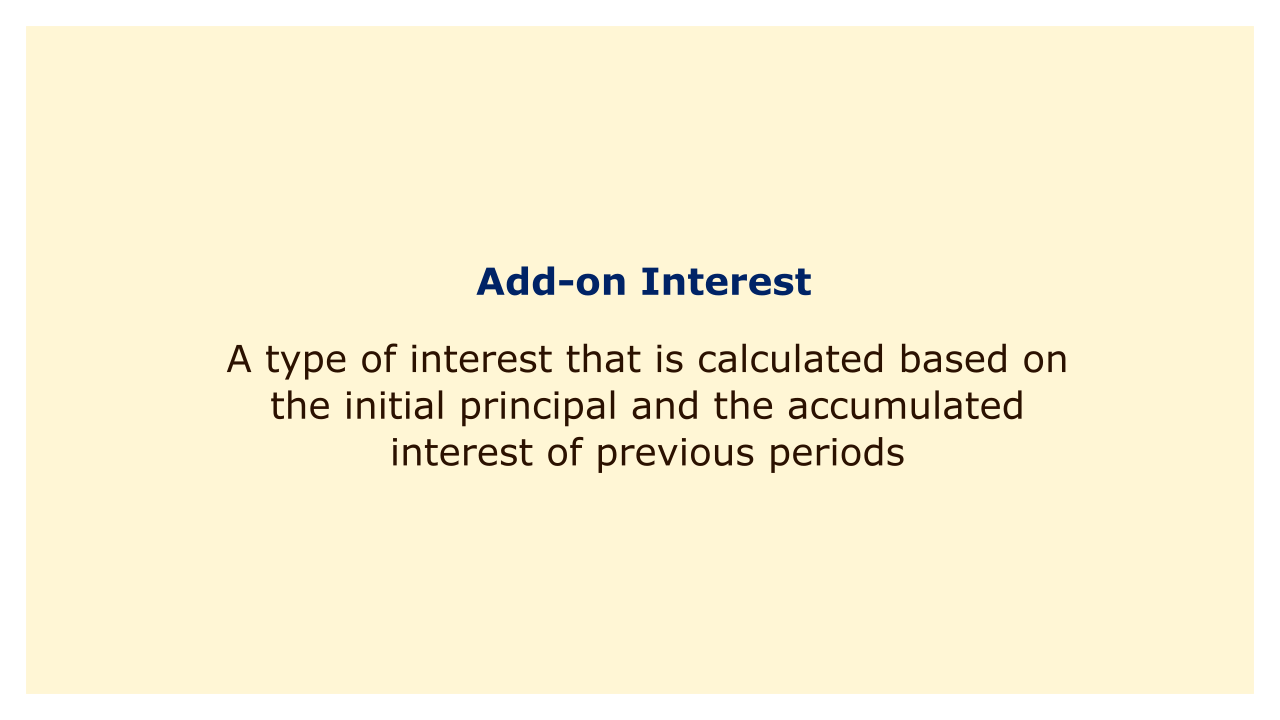Image: Moneybestpal.com

### Add-on interest is a kind of interest that is calculated using the beginning principle and the total interest accrued throughout the course of earlier periods.

In other words, at the end of each period, the interest is added to the principal, and the new, larger principal is then used to compute the interest for the following period. Compound interest is another name for this.

Compared to simple interest, which is calculated using the initial principal, add-on interest is different. Simple interest does not account for interest that is accrued or paid over a period of time. Because of this, when the principle, interest rate, and time periods are the same, basic interest is typically less expensive than add-on interest.

One way to calculate add-on interest is to use the formula:

A = P (1 + rt)

where A is the final amount, P is the initial principal, r is the annual interest rate, and t is the number of years.

For example, suppose you borrow \$1,000 at an add-on interest rate of 10% per year for 3 years. Using the formula, you can find the final amount as follows:

A = 1,000 (1 + 0.1 x 3)

A = 1,000 (1.3)

A = \$1,300

This means that you will have to pay back \$1,300 at the end of 3 years, which includes \$300 of add-on interest.

Another way to calculate add-on interest is to use the compound interest formula:

A = P (1 + r/n)^(nt)

where A is the final amount, P is the initial principal, r is the annual interest rate, n is the number of compounding periods per year, and t is the number of years.

For example, suppose you borrow \$1,000 at an add-on interest rate of 10% per year for 3 years, and the interest is compounded monthly. Using the formula, you can find the final amount as follows:

A = 1,000 (1 + 0.1/12)^(12 x 3)

A = 1,000 (1.0083)^36

A = \$1,347.85

This means that you will have to pay back \$1,347.85 at the end of 3 years, which includes \$347.85 of add-on interest.

As you can see, higher add-on interest rates are achieved when interest is compounded more frequently. This is due to the faster development of the balance caused by more interest being applied to the principal at more frequent periods.

A lot of loans, including mortgages, auto loans, and student loans, include supplemental interest. Understanding how supplemental interest operates, how it affects your payments, and how it impacts your overall borrowing costs is crucial. To analyze several situations and identify the best choice for your circumstances, you can utilize online calculators or spreadsheets.
Tags